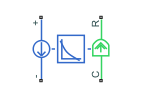# Generic Rotary Actuator

Generic rotary actuator driven from DC voltage source or PWM driver

•Libraries:
Simscape / Electrical / Electromechanical / Mechatronic Actuators

## Description

The Generic Rotary Actuator block implements a model of a generic rotary actuator designed to be driven from a DC voltage source or PWM driver. You define torque-speed characteristics in terms of tabulated values for powering the motor at the rated voltage. This functionality allows you to model a motor without referencing an equivalent circuit.

The motor or actuator architecture determines the way in which electrical losses depend on torque. For example, a DC motor has losses that are proportional to the square of the current. As torque is proportional to current, losses are also proportional to mechanical torque. Most motors have an electrical loss term that is proportional to the square of mechanical torque. The Generic Rotary Actuator block calculates this loss term using the Motor efficiency (percent) and Speed at which efficiency is measured parameters that you provide.

Some motors also have a loss term that is independent of torque. An example is a shunt motor where the field winding draws a constant current regardless of load. The Torque-independent electrical losses parameter accounts for this effect.

The motor efficiency is the mechanical power divided by the sum of the mechanical power and both electrical loss terms. The block assumes that the speed at which the motor efficiency is defined is in the motoring quadrant and, therefore, positive.

You can operate the block in the reverse direction by changing the sign of the voltage that you apply. The H-Bridge block, for example, reverses motor direction if the voltage at the REV port is greater than the Reverse threshold voltage parameter. However, if you are using the block in reverse, specify the torque-speed data for forward operation:

• Positive torques and positive speeds in the motoring quadrant.

• Positive torque and negative speeds in the generating counterclockwise quadrant.

• Negative torque and positive speed in the generating clockwise quadrant.

### Model Thermal Effects

You can expose the thermal port to model the effects of losses that convert power to heat. To expose the thermal port, set the Modeling option parameter to either:

• `No thermal port` — The block does not contain a thermal port.

• `Show thermal port` — The block contains one thermal conserving port.

For more information about using thermal ports in actuator blocks, see Simulating Thermal Effects in Rotational and Translational Actuators.

## Assumptions and Limitations

• The torque-speed curve data corresponds only to the rated voltage, so the block produces accurate results only when driven by plus or minus the rated voltage.

• In this block requires, you must provide torque-speed data for the full range over which you use the actuator. To use the actuator in the generating and braking regions, provide additional data outside of the normal motoring region.

• Model behavior is sensitive to torque-speed data. For example, no-load speed is correctly defined and finite only when the data crosses the speed axis.

• To drive the block from the H-Bridge block:

• Do not place any other blocks between the H-Bridge and the Generic Rotary Actuator blocks.

• In the H-Bridge block dialog box, set the Freewheeling mode to ```Via one semiconductor switch and one freewheeling diode```. Selecting `Via two freewheeling diodes` does not set the bridge output voltage to zero when the PWM input signal is low.

• In the H-Bridge, Generic Rotary Actuator, and Controlled PWM Voltage block dialog boxes, ensure that the Simulation mode is the same for all three blocks.

## Ports

### Conserving

expand all

Electrical conserving port associated with the actuator positive terminal.

Electrical conserving port associated with the actuator negative terminal.

Mechanical rotational conserving port associated with the actuator case.

Mechanical rotational conserving port associated with the rotor.

Thermal port.

#### Dependencies

To enable this port, set Modeling option to `Show thermal port`.

## Parameters

expand all

Whether to enable the thermal port of the block and model the effects of losses that convert power to heat.

### Electrical Torque

Specify a vector of speeds, including their units, for your torque-speed data.

Specify a vector of torques, including their units, for your torque-speed data.

Indicate the voltage for which the device you are modeling is rated.

The efficiency that the block uses to calculate torque-dependent electrical losses.

The speed that the block uses to calculate torque-dependent electrical losses.

Fixed electrical loss associated with the actuator when the torque is zero.

If you set the Simulation mode parameter to `PWM`, apply a PWM waveform switching between zero and rated volts to the block electrical terminals. The current drawn from the electrical supply is equal to the amount required to deliver the mechanical power and to compensate for electrical losses. If the applied voltage exceeds the rated voltage, the resultant torque scales proportionately. However, applying anything other than the rated voltage can provide unrepresentative results.

If you set the Simulation mode parameter to `Averaged`, the torque generated in response to an applied voltage ${V}_{av}$ is

`$\frac{{V}_{av}}{{V}_{rated}}×T\left(\omega \right)$`

where $T\left(\omega \right)$ is the torque value at speed $\omega$. The current drawn from the supply is such that the product of the current and ${V}_{av}$ is equal to the average power that is consumed.

### Mechanical

Rotor resistance to change in motor motion. The value can be zero.

Rotor damping. The value can be zero.

### Temperature Dependence

To enable these parameters, set Modeling option to `Show thermal port`.

Resistance temperature coefficient.

The temperature for which the actuator parameters are defined.

### Thermal Port

To enable this parameter, set Modeling option to `Show thermal port`.

The thermal mass is the energy required to raise the temperature by one degree.

## Version History

Introduced in R2009b